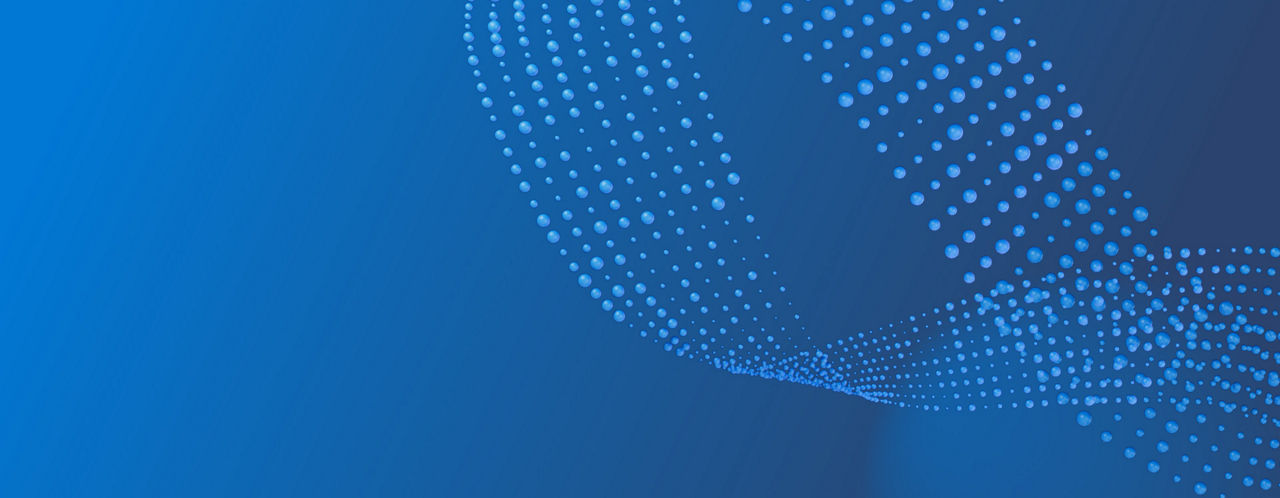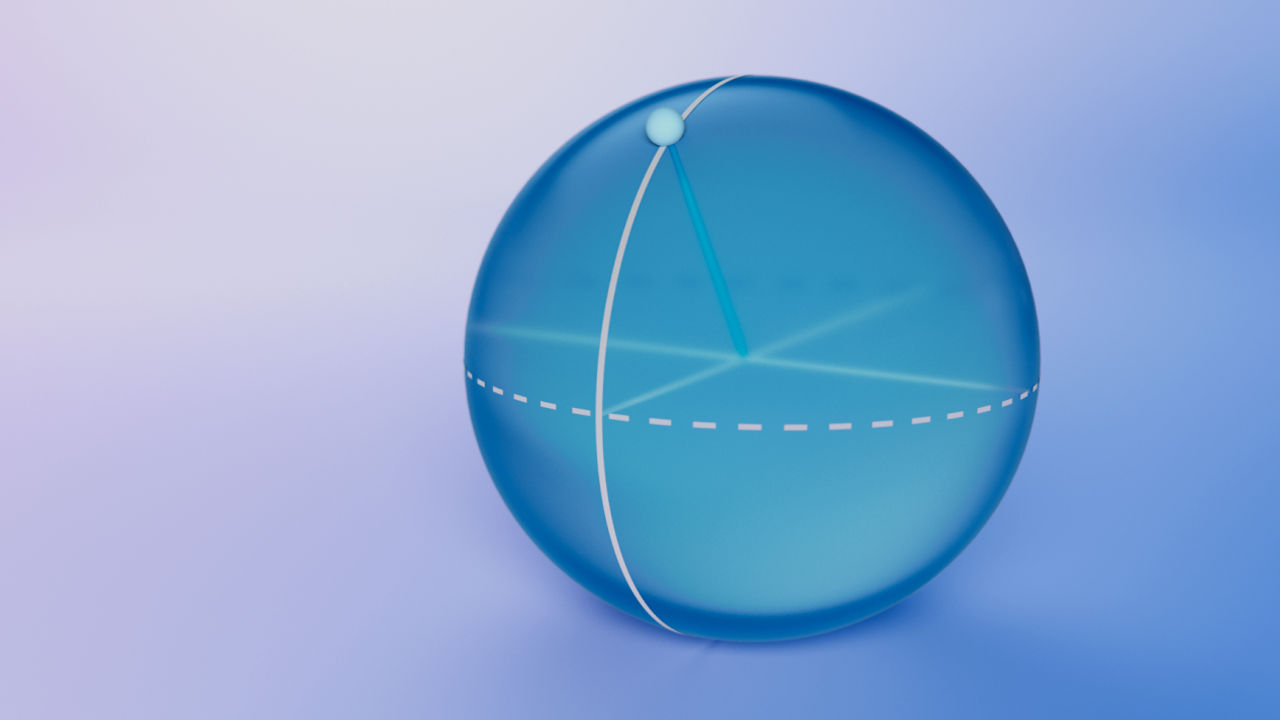# Explore quantum

## The qubit

Just as bits are the fundamental object of information in classical computing, qubits are the fundamental object of information in quantum computing. Although analogous to classical bits, qubits have some important differences. For example, in classical systems bits have a value of 0 or 1 and are physically represented as voltages. For example, in a classical computer a 0 might be represented as 0 volts, while a 1 might be represented by 5 volts. In contrast, a qubit is a two-state quantum-mechanical system. Qubits have been realized using various physical systems, such as electrons, ions or photons. When using an electron, a quantum computing system may use the electron’s spin to represent states, with spin-up representing 1 and spin-down representing 0. If using photons, the system might use polarization to represent states, with vertical polarization representing 1 and horizontal polarization representing 0.### How is a qubit different from a classical bit?

Bits in classical computers can only be in a 1 state or a 0 state. Qubits, however, are probabilistic and can exist in a quantum mechanical state of superposition, in which the qubit will be in both the 0 and 1 states simultaneously. (More specifically, a qubit in superposition exists in a linear combination of the two states.) Because of superposition, qubits can represent more information than a classical bit. For example, two classical bits can represent four possible states (00, 01, 10, and 11), while two qubits can represent any combination of these four states.

Qubits can also be entangled with each other. Entanglement is a property of qubits that allows their states to be correlated in a way that is not possible with classical bits. When two qubits are entangled, their states become linked in such a way that the state of one qubit depends on the state of the other. For example, suppose we have two qubits that are entangled. When measured, we find that one of the qubits is in the state |0⟩. This will cause the other qubit to collapse into a state that is correlated with the first qubit. Entanglement is an important property of quantum computing because it allows quantum computers to perform certain calculations much faster than classical computers.

### Suggested Topics

#### Types of qubits

Learn about different qubit implementations, from super conducting, to ion trap, to topological qubits

#### Topological qubits

Microsoft believes topological qubits are the key to unlocking scaled quantum computing. Learn more about our unique approach

#### Quantum math

Learn about the mathematics behind quantum computing, and how it is used to describe the behavior of qubits and quantum gates

#### Dirac notation

Learn about Dirac notation, also known as bra-ket notation, and how it is used to represent quantum states and operations